# 基本数据类型

## 集算器中的数据类型

Ø  整数

32位整数，即-231~231-1之间的整数，其取值范围-2147483648~2147483647，可用类型转换函数int()将其它类型数据转换为32位整数。

Ø  长整数

64位整数，即-263~263-1之间的整数，比整数类型的取值范围更大，可用类型转换函数long()将其它类型数据转换为长整数。

 A 1 =123456789L*1000 2 =123456789*1000 3 =12345678900*10 4 0x00FF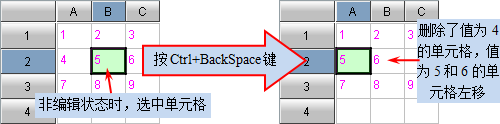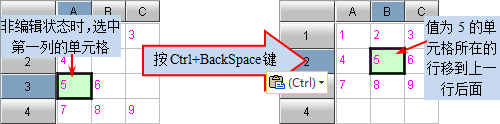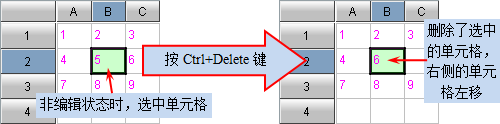A1A3中的计算结果可以发现，在进行某一步运算时，当参与运算的操作数之一为长整数，结果就是长整数。在集算器中，数值类的数据在显示时都会靠右对齐，整数会用蓝色显示，而长整数会用黑色显示。

Ø  浮点数

64位浮点数，这是集算器中最常用的数据类型，涉及小数的运算基本都是用它来计算的，可用类型转换函数float()将其它类型数据转换为浮点数。由于浮点数是用二进制规则存储数据的，因此在计算中有可能出现误差。

 A 1 43.41 2 -4.45E13 3 3% 4 =float(54)

A1,A2,A3A4中的值如下：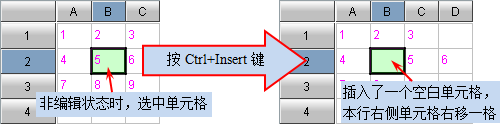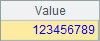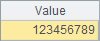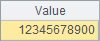Ø  长实数

 A 1 5.2 2 =A1*A1 3 =decimal("5.2") 4 =A3*A3

A1中为浮点数5.2A3中将字符串"5.2"转换为长实数。A2A4中计算的结果如下：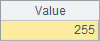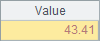Ø  实数

Ø  布尔型

 A 1 true 2 false 3 =4>2 4 =13%3==0

A1,A2,A3A4中的结果如下：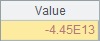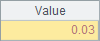Ø  字符串

 A 1 Amy 2 ="State:\tTX" 3 ="Texas" "Dallas" 4 =A1+3.14 5 =A1/3.14

A1,A2,A3,A4A5中的结果如下：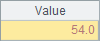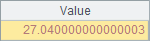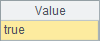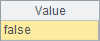Ø  日期/时间

 A 1 2014-10-5 2 22:15:30 3 =date("2014" "-10" "-5")

A1,A2A3中的结果如下：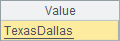Ø  排号

 A B 1 =k(1111:2) =A1.len() 2 =A1{1} =A2.len() 3 =k(1111:4) =A3.len() 4 =[A3{1},A3{2},A3{3},A3{4}] =k(0,0,4,87) 5 =A3==B4 =A3==A1 6 =k(111,0,0,4,0,0,0,87) =k(133,255,0,128,0,0,0,0) 7 =A6

A1中用k(n:l) 函数生成排号，使用整数1111来生成2个字节的排号数据。可以用k.len()来查看排号数据的字节数，A1B1中的值如下：k{n}可以取出排号数据的某个字节来生成新的排号数据，A2中取出排号A1的第一个字节构成单字节的排号数据，A2B2中结果如下：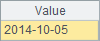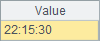A3中，同样用整数1111生成排号数据，但占用4个字节。A4中查看A3各个字节的数据情况。也可以直接用k(ni,…) 指定各个字节的值来生成排号数据。A3B3A4B4中的结果如下：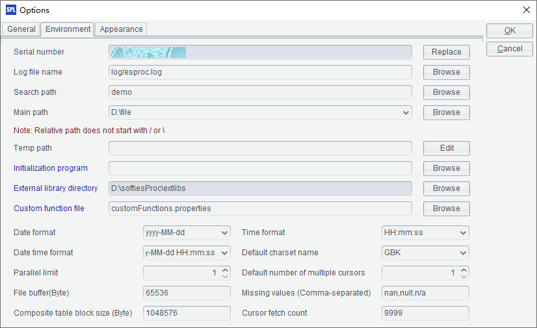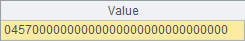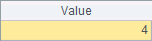A5B5中，用A3中的排号与B4A1中的排号作对比，可以发现，B4中生成的排号与A3中是相同的，而不同长度的排号数据，即使显示的值相同，也是不同的排号数据。A5B5中的比较结果如下：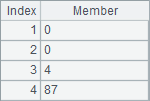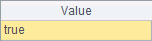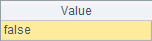## 数据类型的判断

Ø  ifnumber(x)

Ø  ifstring(x)

Ø  ifdate(x)

Ø  iftime(x)

 A 1 =ifnumber(3.5) 2 =ifstring(now())

A1中，3.5是浮点数，属于实数。A2now()函数计算当前日期时间，结果不是字符串。A1A2中的结果如下：